This page will provide an overview of the algorithm and describe how it works and how it can be used. To do this, let's start by briefly describing random forests.

## Random forests

Random forests are known to produce accurate predictions especially in settings where the number of features p is close to or higher than the number of observations n (Biau & Scornet, 2016). Let's start by explaining the building blocks of random forests: decision trees. As an example, we take Haberman's Survival Data Set (see the Appendix below for more code details):

begin
using CairoMakie
using CategoricalArrays: categorical
using CSV: CSV
using DataFrames
using DecisionTree: DecisionTree
using EvoTrees: EvoTreeClassifier
using MLJDecisionTreeInterface: DecisionTreeClassifier
using MLJ: CV, MLJ, Not, PerformanceEvaluation, auc, fit!, evaluate, machine
using StableRNGs: StableRNG
using SIRUS
using Statistics: mean, std
end
data = _haberman()
ageyearnodessurvival
130.01964.01.01
230.01962.03.01
330.01965.00.01
431.01959.02.01
531.01965.04.01
633.01958.010.01
733.01960.00.01
834.01959.00.00
934.01966.09.00
1034.01958.030.01
...
30683.01958.02.00
X = data[:, Not(:survival)];
y = data.survival;

This dataset contains observations from a study with patients who had breast cancer. The survival column contains a 0 if a patient has died within 5 years and 1 if the patient has survived for at least 5 years. The aim is to predict survival based on the age, the year in which the operation was conducted and the number of detected axillary nodes.

Via MLJ.jl, we can fit multiple decision trees on this dataset:

tree_evaluations = let
model = DecisionTreeClassifier(; max_depth=2, rng=_rng())
_evaluate(model, X, y)
end;

This has fitted various trees to various subsets of the dataset via cross-validation. Here, I've set max_depth=2 to simplify the fitted trees which makes the tree more easily explainable. Also, for our small dataset, this forces the model to remain simple so it likely reduces overfitting. Let's look at the first tree:

let
tree = tree_evaluations.fitted_params_per_fold.raw_tree
_io2text() do io
DecisionTree.print_tree(io, tree; feature_names=names(data))
end
end
Feature 3: "nodes" < 2.5 ?
├─ Feature 1: "age" < 79.5 ?
├─ 2 : 151/178
└─ 1 : 1/1
└─ Feature 1: "age" < 43.5 ?
├─ 2 : 16/20
└─ 1 : 42/76


What this shows is that the first tree decided that the nodes feature is the most helpful in deciding who will survive for 5 more years. Next, if the nodes feature is below 2.5, then age will be selected on. If age < 79.5, then the model will predict the second class and if age ≥ 79.5 it will predict the first class. Similarly for age < 43.5. Now, let's see what happens for a slight change in the data. In other words, let's see how the fitted model for the second split looks:

let
tree = tree_evaluations.fitted_params_per_fold.raw_tree
_io2text() do io
DecisionTree.print_tree(io, tree; feature_names=names(data))
end
end
Feature 3: "nodes" < 2.5 ?
├─ Feature 1: "age" < 77.0 ?
├─ 2 : 147/175
└─ 1 : 2/2
└─ Feature 1: "age" < 43.5 ?
├─ 2 : 18/21
└─ 1 : 41/77


This shows that the features and the values for the splitpoints are not the same for both trees. This is called stability. Or in this case, a decision tree is considered to be unstable. This instability is problematic in situations where real-world decisions are based on the outcome of the model. Imagine using this model for the selecting which students are allowed to enter some university. If the model is updated every year with the data from the last year, then the selection criteria would vary wildly per year. This instability also causes accuracy to fluctuate wildly. Intuitively, this makes sense: if the model changes wildly for small data changes, then model accuracy also changes wildly. This intuitively also implies that the model is more likely to overfit. This is why random forests were introduced. Basically, random forests fit a large number of trees and average their predictions to come to a more accurate prediction. The individual trees are obtained by restricting the observations and the features that the trees are allowed to use. For the restriction on the observations, the trees are only allowed to see partial_sampling * n observations. In practise, partial_sampling is often 0.7. The restriction on the features is defined in such a way that it guarantees that not every tree will take the same split at the root of the tree. This makes the trees less correlated (James et al., 2021; Section 8.2.2) and, hence, more accurate.

Unfortunately, these random forests are hard to interpret. To interpret the model, individuals would need to interpret hundreds to thousands of trees containing multiple levels. Alternatively, methods have been created to visualize these uninterpretable models (for example, see Molnar (2022); Chapters 6, 7 and 8). The most promising one of these methods are Shapley values and SHAP. These methods show which features have the highest influence on the prediction. See my blog post on Random forests and Shapley values for more information. Knowing which features have the highest influence is nice, but they do not state exactly what feature is used and at what cutoff. Again, this is not good enough for selecting students into universities. For example, what if the government decides to ask for details about the selection? The only answer that you can give is that some features are used for selection more than others and that they are on average used in a certain direction. If the government asks for biases in the model, then these are impossible to report. In practice, the decision is still a black-box. SIRUS solves this by extracting easily interpretable rules from the random forests.

## Rule-based models

Rule-based models promise much greater interpretability than random forests. Instead of returning a large number of trees, rule-based models return a set of rules. Each rule can be interpreted on its own and the final model aggregates these rules by summing the prediction of each rules. For example, one rule can be:

if nodes < 4.5 then chance of survival is 0.6 and if nodes ≥ 4.5 then chance of survival is 0.4.

Note that these rules can be extracted quite easily from the decision trees. For splits on the second level of the tree, the rule could look like:

if nodes < 4.5 and age < 38.5 then chance of survival is 0.8 and otherwise the chance of survival is 0.4.

When applying this extracting of rules to a random forest, there will be thousands of rules. Next, via some heuristic, the most important rules can be localized and these rules then result in the final model. See, for example, RuleFit (Friedman & Popescu, 2008). The problem with this approach is that they are fitted on the unstable decision trees that were shown above. As an example, on time the tree splits on age < 43.5 and another time on age < 44.5.

## Tree stabilization

In the papers which introduce SIRUS, Bénard et al. (2021a, 2021b) proof that their algorithm is stable and that the other algorithms are not. They achieve their stability by restricting the location at which the splitpoints can be chosen. To see how this works, let's look at the age feature on its own.

nodes = sort(data.age);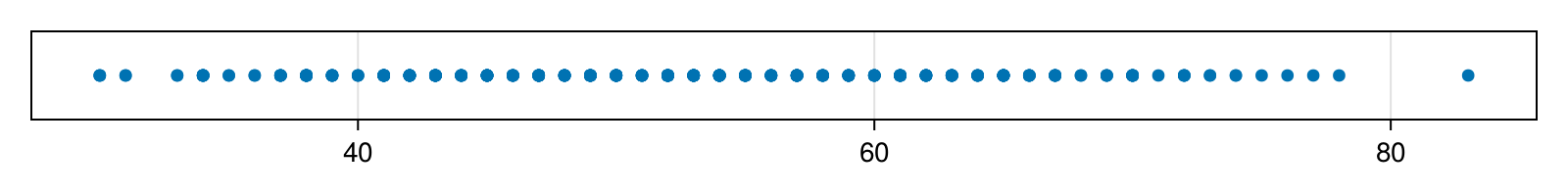The default random forest algorithm is allowed to choose any location inside this feature to split on. To avoid having to figure out locations by itself, the algorithm will choose on of the datapoints as a split location. So, for example, the following split indicated by the red vertical line would be a valid choice: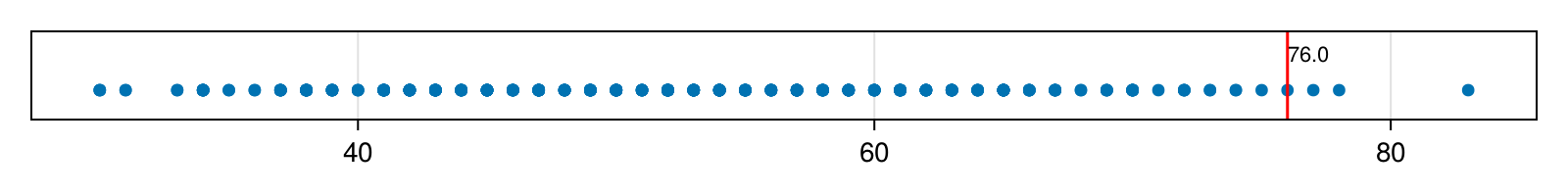But what happens if we take a random subset of the data? Say, we take the following subset of length 0.7 * length(nodes):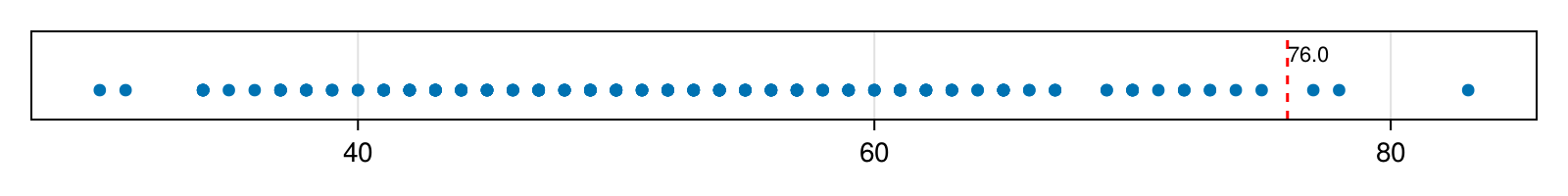Now, the algorithm would choose a different location and, hence, introduce instability. To solve this, Bénard et al. decided to limit the splitpoints that the algorithm can use to split to data to a pre-defined set of points. For each feature, they find q empirical quantiles where q is typically 10. Let's overlay these quantiles on top of the age feature: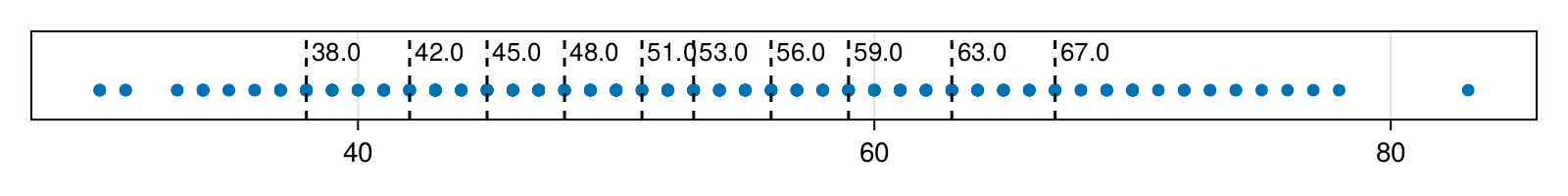Next, let's see where the cutpoints are when we take the same random subset as above: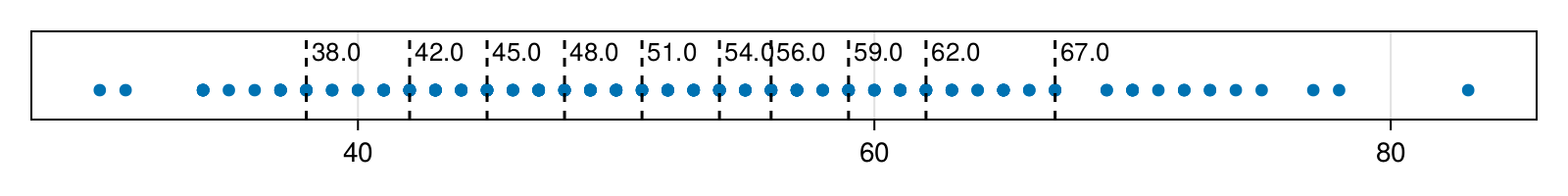As can be seen, many cutpoints are at the same location as before. Furthermore, compared to the unrestricted range, the chance that two different trees who see a different random subset of the data will select the same cutpoint has increased dramatically.

The benefit of this is that it is now quite easy to extract the most important rules. Rule extraction consists of simplifying them a bit and ordering them by frequency of occurrence. Let's see how accurate this model is.

## Benchmarks

Let's compare the following models:

• Decision tree (DecisionTreeClassifier)

• Stabilized random forest (StableForestClassifier)

• SIRUS (StableRulesClassifier)

• EvoTrees (EvoTreeClassifier)

The latter is a gradient boosting model. See the Appendix for more details about these results.

e1 = let
model = DecisionTreeClassifier
hyperparameters = (; rng=_rng(), max_depth=2)
_evaluate(model, hyperparameters, X, y)
end;
e2 = let
model = StableRulesClassifier
hyperparameters = (; rng=_rng(), q=4, max_depth=2, max_rules=8)
_evaluate(model, hyperparameters, X, y)
end;
e3 = let
model = StableRulesClassifier
hyperparameters = (; rng=_rng(), q=4, max_depth=2, max_rules=25)
_evaluate(model, hyperparameters, X, y)
end;
e4 = let
model = StableRulesClassifier
hyperparameters = (; rng=_rng(), q=4, max_depth=1, max_rules=25)
_evaluate(model, hyperparameters, X, y)
end;
e5 = let
model = StableForestClassifier
hyperparameters = (; rng=_rng(), q=4, max_depth=2)
_evaluate(model, hyperparameters, X, y)
end;
e6 = let
model = EvoTreeClassifier
hyperparameters = (; rng=_rng())
_evaluate(model, hyperparameters, X, y)
end;
e7 = let
model = EvoTreeClassifier
hyperparameters = (; rng=_rng(), max_depth=2)
_evaluate(model, hyperparameters, X, y)
end;
#hideall
results = let
df = DataFrame(getproperty.([e6, e7, e1, e5, e3, e2, e4], :row))
df[!, :Interpretability] = ["Low", "Low", "High", "Low", "High", "High", "High"]
df[!, :Stability] = ["High", "High", "Low", "High", "High", "High", "High"]
df[!, :AUC] = map(df.AUC) do score
text = string(score)
length(text) < 4 ? text * '0' : text
end
df[:, :Model] = map(zip(df.Model, df.Hyperparameters)) do (model, param)
text = string(model, param)
text = replace(text, " = " => "=")
text = replace(text, ",)" => ")")
text = replace(text, "(;)" => "()")
end
select!(df, Not(:Hyperparameters))
for col in names(df)
df[!, col] = Base.Text.(df[:, col])
end
rename!(df, :se => "1.96*SE")
end
ModelAUC1.96*SEInterpretabilityStability
1EvoTreeClassifier()0.620.07LowHigh
2EvoTreeClassifier(max_depth=2)0.680.04LowHigh
3DecisionTreeClassifier(max_depth=2)0.620.06HighLow
4StableForestClassifier(q=4, max_depth=2)0.700.06LowHigh
5StableRulesClassifier(q=4, max_depth=2, max_rules=25)0.700.07HighHigh
6StableRulesClassifier(q=4, max_depth=2, max_rules=8)0.700.08HighHigh
7StableRulesClassifier(q=4, max_depth=1, max_rules=25)0.690.07HighHigh

As can be seen, the score of the stabilized random forest (StableForestClassifier) is comparable to the gradient boosted Evo Trees (EvoTreesClassifier), but both are not interpretable since that requires interpreting thousands of trees. With the rule-based classifier (StableRulesClassifier), a small amount of predictive performance can be traded for high interpretability. Note that the rule-based classifier may actually be more accurate in practice because verifying and debugging the model is much easier.

Regarding the hyperparameters, tuning max_rules and max_depth has the most effect. max_rules specifies the number of rules to which the random forest is simplified. Setting to a high number such as 999 makes the predictive performance similar to that of a random forest, but also makes the interpretability as bad as a random forest. Therefore, it makes more sense to truncate the rules to somewhere in the range 5 to 40 to obtain accurate models with high interpretability. max_depth specifies how many levels the trees have. For larger datasets, max_depth=2 makes the most sense since it can find more complex patterns in the data. For smaller datasets, max_depth=1 makes more sense since it reduces the chance of overfitting. It also simplifies the rules because with max_depth=1, the rule will contain only one subclause (for example, "if A then ...") versus two subclauses (for example, "if A & B then ..."). In some cases, model accuracy can be improved by increasing n_trees. The higher this number, the more trees are fitted and, hence, the higher the chance that the right rules are extracted from the trees.

## Interpretation

Finally, let's interpret the rules that the model has learned. Since we know that the model performs well on the cross-validations, we can fit our preferred model on the complete dataset:

fitresult = let
model = StableRulesClassifier(; q=4, max_depth=1, max_rules=8, rng=_rng())
mach = machine(model, X, y)
fit!(mach)
mach.fitresult
end
StableRules model with 8 rules:
if X[i, :nodes] < 7.0 then 0.15 else 0.099 +
if X[i, :nodes] < 2.0 then 0.097 else 0.063 +
if X[i, :year] < 1959.0 then 0.004 else 0.005 +
if X[i, :age] < 42.0 then 0.041 else 0.034 +
if X[i, :age] < 55.0 then 0.053 else 0.064 +
if X[i, :year] < 1966.0 then 0.08 else 0.092 +
if X[i, :age] < 62.0 then 0.089 else 0.104 +
if X[i, :year] < 1964.0 then 0.062 else 0.053
and 2 classes: [0, 1].
Note: showing only the probability for class 1 since class 0 has probability 1 - p.


The interpretation of the fitted model is as follows. The model has learned 8 rules for this dataset. For making a prediction for some value at row i, the model will first look at [i, :nodes] < 7.0. If the current value satisfies this rule, then the number after then is chosen and otherwise the number after else. The then or else outcome is chosen for all the rules and, finally, the outcomes are summed to obtain the final prediction.

## Plot

Since our rules are relatively simple with only a binary outcome and only one subclause in each rule (because of max_depth=1), the following figure is a way to visualize the obtained rules per fold. For multiple subclauses, I would not know how to visualize the rules. Also, this plot is probably not perfect; let me know if you have suggestions.

This figure shows the model uncertainty by visualizing the obtained models for different cross-validation folds. The subfigure on the left shows how the fitted rules affect the outcome. A point on the left means that a lower value for the data is related to higher outcome (i.e., a higher chance of survival in this dataset) whereas a point on the right is means that a higher value for the data is related to a higher outcome. The subfigure on the right shows the thresholds used by the rules in the cross-validation folds via the vertical lines. In the background, the histograms show the data.

For example, for the nodes, it can be seen that all rules (fitted in the different cross-validation folds) base their decision on whether the nodes are below, roughly, 5. Next, the left side indicates in which direction this effect works. More specifically, the individuals who had less than 5 nodes are more likely to survive, according to the model. The sizes of the dots indicate the weight that the rule has, so a bigger dot means that a rule plays a larger role in the final outcome. These dots are sized in such a way that a doubling in weight means a doubling in surface size.

function _odds_plot(models::Vector{<:StableRules}, feat_names::Vector{String})
w, h = (1000, 300)
fig = Figure(; size=(w, h))
grid = fig[1, 1:2] = GridLayout()

@assert feat_names == sort(unique(feat_names))

probability_for_class_1(probs::Vector) = last(probs)::Float64
# Gets the feature importances in order of importance.
importances = feature_importances(models, feat_names)

# Create a row in the plot for each feature.
for (i, name_importance) in enumerate(importances)
feat_name, importance = name_importance
let
yticks = (1:1, [feat_name])
ax1 = Axis(grid[i, 1:2]; yticks)
barplot!(ax1, [importance]; direction=:x)
xlims!(ax1, 0, 1.05 * first(importances).importance)
hidexdecorations!(ax1)
end
ax2 = Axis(grid[i, 3:4])
ax3 = Axis(grid[i, 5:6])
vlines!(ax2, ; color=:gray, linestyle=:dash)
xlims!(ax2, -1, 1)

unpacked_rules = unpack_models(models, feat_name)::Vector{NamedTuple}
# Create a dot and line for each rule that mentions the current feature.
for unpacked_rule::NamedTuple in unpacked_rules
left = probability_for_class_1(unpacked_rule.then)
right = probability_for_class_1(unpacked_rule.otherwise)
value = unpacked_rule.splitval
ratio = log(right / left)
# area = πr²
markersize = 50 * sqrt(unpacked_rule.weight / π)
scatter!(ax2, [ratio], ; color=:black, markersize)

vlines!(ax3, unpacked_rule.splitval; color=:black, linestyle=:dash)
end

# Show a histogram in the background.
hist!(ax3, data[:, feat_name]; scale_to=1)

hidexdecorations!(ax2)
hideydecorations!(ax3)
hidexdecorations!(ax3; ticks=false, ticklabels=false)
end

return fig
end;
let
models = getproperty.(e4.e.fitted_params_per_fold, :fitresult)
_odds_plot(models, sort(names(X)))
end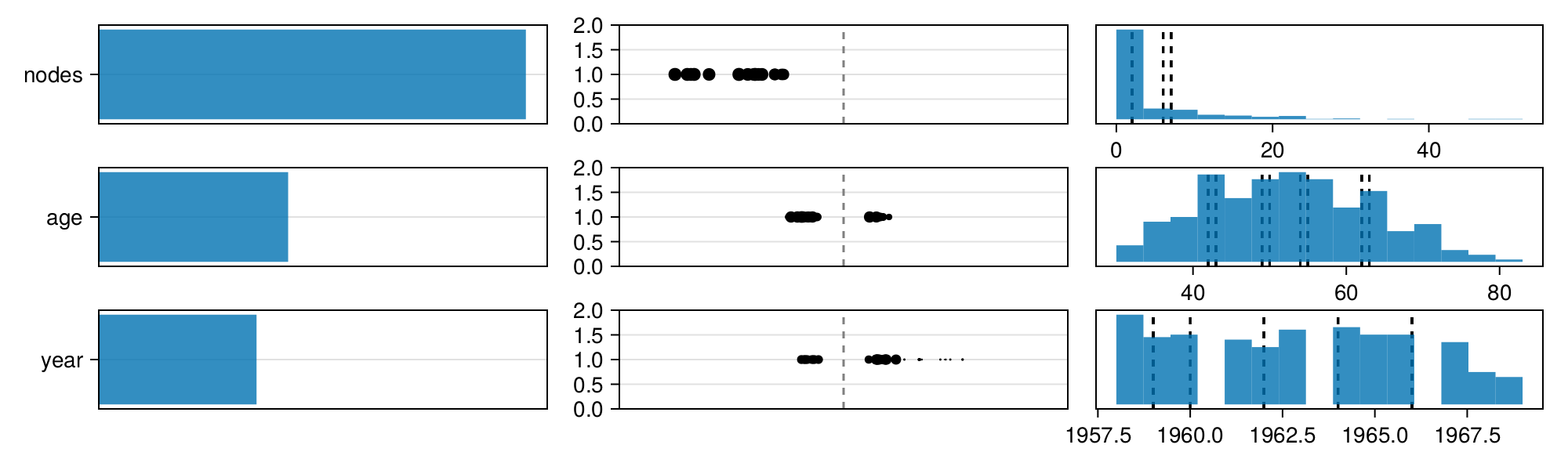What this plot shows is that the nodes feature scored highest in the feature_importance function, which means that nodes is estimated as the feature with the most predictive power. This makes sense because it says that the fewer auxiliary nodes are detected, the higher the chance of survival. Furthermore, a lower age seems to also be slightly related to a higher chance of survival, but this effect has a much lower importance (size of bar in leftmost axis) and also differs much more between the different train-test splits (dots in middle axis). The year in which the operation was conducted appears to not have a serious effect on the survivability and the plot shows this via a small feature importance (leftmost axis), a high variability on the direction of that feature (dots in middle axis) and the high variability on the split points (rightmost axis).

## Practical applications

As shown in the previous sections, the model satisfies two things:

1. It shows a good predictive performance in the model evaluations. The performance is slightly lower than more complex models, but this tradeoff can be worth it because the rule-based model is interpretable.

2. The fitted model makes theoretical sense. As shown in the visualization, the nodes and age features are the most important for prediction and both features are used in the expected way.

Since the model shows good performance and makes theoretical sense, we can be reasonably sure that the model will generalize to new data in a similar context. Next, the model can be applied by fitting it on the full dataset and brining it to a real-world setting.

Note that unlike the random forests and gradient boosted trees, each decision that the model makes can be fully explained. All rules can be read stand-alone and interpreted. For example, when trying to interpret a random forest, it will only report feature importances. For the Haberman dataset, we would know more than nodes is negatively associated and age too. With the rule-based model, we can say exactly at which number of nodes and at which age the model decides to split the data between likely to survive or not survive.

## Conclusion

Compared to decision trees, the rule-based classifier is more stable, more accurate and similarly easy to interpret. Compared to the random forest, the rule-based classifier is only slightly less accurate, but much easier to interpret. Due to the interpretability, it is likely easier to verify the model and therefore the rule-based classifier will be more accurate in real-world settings. This makes rule-based highly suitable for many machine learning tasks.

## Appendix

function _plot_cutpoints(data::AbstractVector)
fig = Figure(; size=(800, 100))
ax = Axis(fig[1, 1])
cps = Float64.(unique(cutpoints(data, 10)))
scatter!(ax, data, fill(1, length(data)))
vlines!(ax, cps; color=:black, linestyle=:dash)
textlocs = [(c, 1.1) for c in cps]
for cutpoint in cps
annotation = string(round(cutpoint; digits=2))::String
text!(ax, cutpoint + 0.2, 1.08; text=annotation, fontsize=13)
end
ylims!(ax, 0.9, 1.2)
hideydecorations!(ax)
return fig
end;
_rng(seed::Int=1) = StableRNG(seed);
function _io2text(f::Function)
io = IOBuffer()
f(io)
s = String(take!(io))
return Base.Text(s)
end;
function _evaluate(model, X, y; nfolds=10)
resampling = CV(; nfolds, shuffle=true, rng=_rng())
evaluate(model, X, y; acceleration, verbosity=0, resampling, measure=auc)
end;
function register_haberman()
name = "Haberman"
message = "Slightly modified copy of Haberman's Survival Data Set"
checksum = "a7e9aeb249e11ac17c2b8ea4fdafd5c9392219d27cb819ffaeb8a869eb727a0f"
end;
function _haberman()
register_haberman()
path = joinpath(dir, "haberman.csv")
df[!, :survival] = categorical(df.survival)
# Need Floats for the LGBMClassifier.
for col in [:age, :year, :nodes]
df[!, col] = float.(df[:, col])
end
return df
end;
_filter_rng(hyper::NamedTuple) = Base.structdiff(hyper, (; rng=:foo));
_pretty_name(modeltype) = last(split(string(modeltype), '.'));
function _evaluate(modeltype, hyperparameters, X, y)
model = modeltype(; hyperparameters...)
e = _evaluate(model, X, y)
row = (;
Model=_pretty_name(modeltype),
Hyperparameters=_hyper2str(_filter_rng(hyperparameters)),
AUC=_score(e),
se=round(only(MLJ.MLJBase._standard_errors(e)); digits=2)
)
(; e, row)
end;
_hyper2str(hyper::NamedTuple) = hyper == (;) ? "(;)" : string(hyper)::String;
function _score(e::PerformanceEvaluation)
return round(only(e.measurement); digits=2)
end;